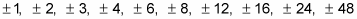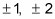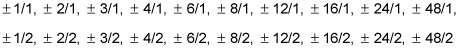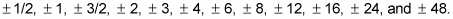##### Basic Math & Pre-Algebra All-in-One For Dummies (+ Chapter Quizzes Online)When you look for all the possible rational roots of any polynomial, the first step is to use the rational root theorem to list them all.

The rational root theorem says that if you take all the factors of the constant term in a polynomial and divide by all the factors of the leading coefficient, you produce a list of all the possible rational roots of the polynomial. However, keep in mind that you’re finding only the rational ones, and sometimes the roots of a polynomial are irrational. Some of your roots may also be imaginary, but save those until the end of your search.

For example, consider the equation f(x) = 2x4 – 9x3 – 21x2 + 88x + 48. The constant term is 48, and its factors are as follows:The leading coefficient is 2, and its factors are as follows:So the list of possible real roots includes the following:Thankfully, these roots all simplify to